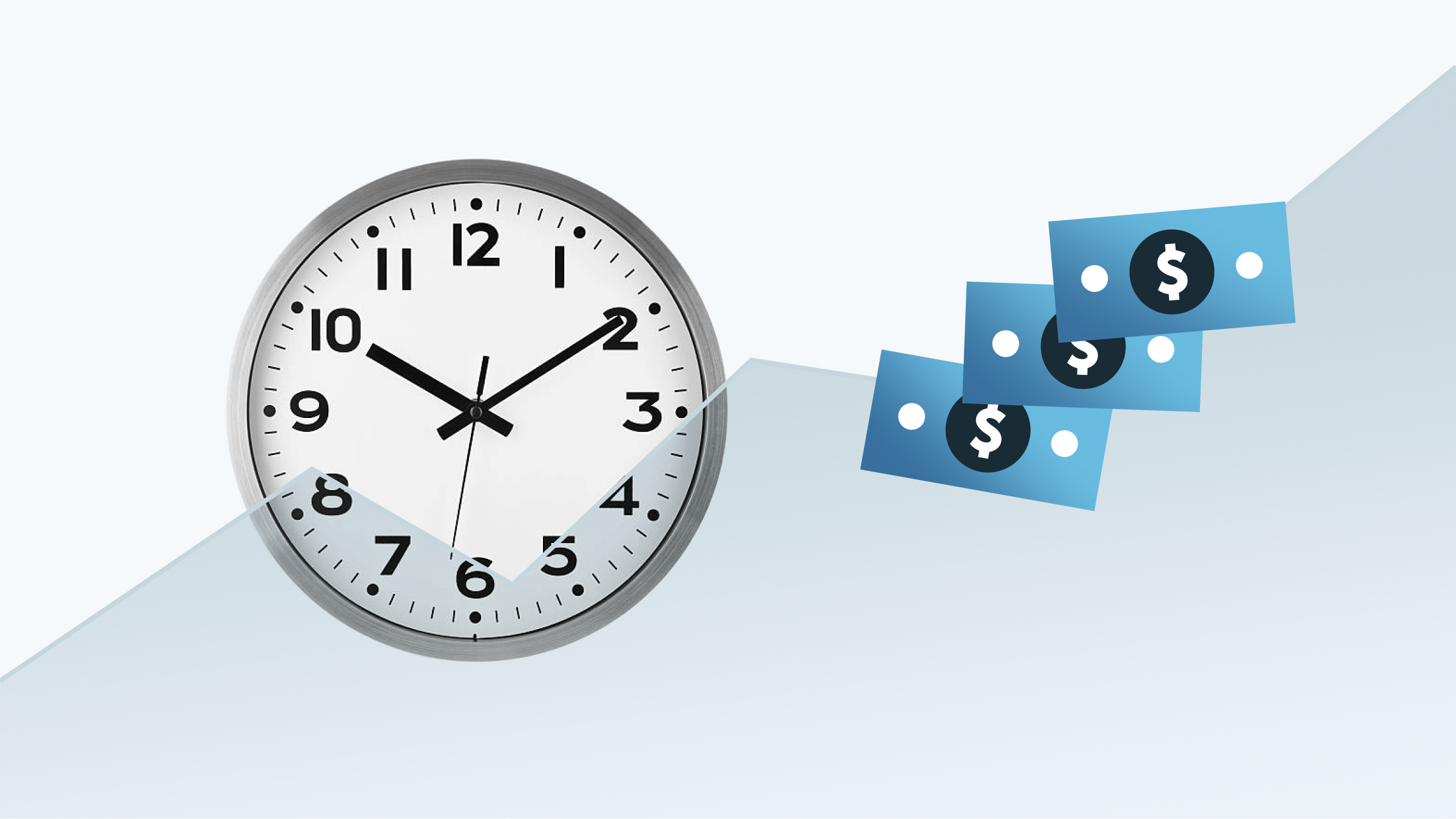ALL BLOGS
Education

# Breaking Down IRRInternal Rate of Return (IRR) is one of the most frequently used metrics in real estate valuation. It offers a handy way to compare assets with different return profiles because it incorporates the concept of time value of money: the idea that a dollar today is worth more than a dollar one year, or one decade, from now. Let’s break it down.

Here are the variables that we need to calculate an asset’s IRR:

• t = The number of time periods (often measured in years) that we expect to hold the asset
• Ct = The net cash flow we earn from the asset in each year
• C0 = The initial cost of the asset

Packaging those variables together, we can then solve for IRR. This is the formula we use:

In mathematical terms, IRR is the rate that sets the net present value of all future cash flows equal to 0. In plain speak - it’s the annual rate of return that we expect to earn from an asset.

Let’s run through a hypothetical example:

Say we’re considering the purchase of a multifamily asset in Brooklyn for \$20,000,000. We expect \$2,000,000 per year in rental income. We expect to sell the property after four years for \$28,000,000. For the sake of simplicity, let’s assume that we’re not paying interest on a loan and that no capital will be spent on building maintenance or taxes.

In this simplified example, the cash flow over four years would look like this:

Year 0 = C1 in our equation = -\$20,000,000 (our initial cash investment)

Year 1 = C1 in our equation = \$2,000,000 (rental income)

Year 2 = C2 in our equation = \$2,000,000 (rental income)

Year 3 = C3 in our equation = \$2,000,000 (rental income)

Year 4 = C4 in our equation = \$2,000,000 (rental income) + \$28,000,000 (sale price) = \$30,000,000

We can then plug these values into our IRR formula:

NPV = 0 = 2,000,000/(1+IRR)1 + 2,000,000/(1+IRR)2 + 2,000,000/(1+IRR)3 + 30,000,000/(1+IRR)4 - 20,000,000

Solving for IRR in this equation, we get a healthy expected return of 17.703%. Want to check our math? There are plenty of free calculators online that will crunch the numbers for you.

Note that in the example above, we made some big assumptions. We estimated a sale price of \$28,000,000 at the end of the four year period, which is dependent on robust economic growth in our Brooklyn neighborhood and a major increase in demand for apartment units. Our example also doesn’t account for the possibility of expensive repairs, apartment vacancies, and other costs that will eat into our annual returns. Rest assured that all these factors are carefully considered by our Real Estate team when we evaluate a property for the LEX platform - the IRR model is just the start!

LEX Markets does not provide tax, legal or accounting advice. Potential investors are encouraged to consult with professional tax, legal, and financial advisors before making any investment into a securities offering. This investment may not be suitable for all investors. Distributions and liquidity not guaranteed. Property performance and performance of property tenants not guaranteed. Diversification does not eliminate the risk of experiencing investment loss.

November 15, 2022